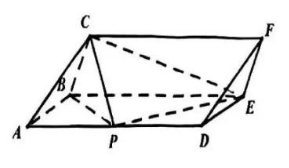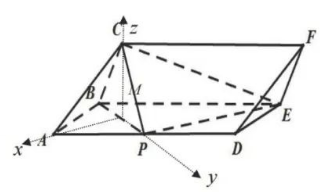(1) 求证: $P E \perp$ 平面 $B C P$;
(2) 求平面 $E C P$ 与平面 $C D P$ 夹角的余弦值.【答案】 【解析】 (1) 取 $B P$ 中点 $M$, 连接 $A M 、 C M$, 易知 $A M \perp B P$ 且 $C M \perp B P$, 因此 $B P \perp$ 平面 $A C M$, 因为 $B P \subset$ 平面 $A B P$, 所以平面 $A C M \perp$ 平面 $A B P$, 因为平面 $A C M \cap$ 平面 $A B P=A M$,

(2) 由 (1) 可知 $M P 、 M C 、 M A$ 两两垂直, 以 $M$ 为原点, $M A$ 所在直线为 $x$ 轴, $M P$ 所在直线为 $y$ 轴, $M C$ 所在直线为 $z$ 轴建立空间直角坐标系, 所以 $C(0,0, \sqrt{3}), P(0,1,0), D(-\sqrt{3}, 2,0), E(-2 \sqrt{3}, 1,0)$,

$$\overrightarrow{n_1}=(0, \sqrt{3}, 1),$$

$$\text { 则 }\left\{\begin{array} { l } { \vec { n _ { 2 } } \cdot \vec { P C } = 0 } \\ { \vec { n _ { 2 } } \cdot \vec { P D } = 0 } \end{array} \Rightarrow \left\{\begin{array}{l} -y_2+\sqrt{3} z_2=0 \\ y_2-\sqrt{3} x_2=0 \end{array} \text {, 令 } y_2=\sqrt{3} \text { 得 } \overrightarrow{n_2}=(1, \sqrt{3}, 1)\right.\right. \text {, }$$